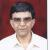true

Take Class 10 Tuition from the Best Tutors

•Affordable fees
•1-1 or Group class
•Flexible Timings
•Verified Tutors

Search inAshish K Sharma
16/06/2022 00

Q: The numerator of a fraction is 3 less than the denominator. If 2 is added to

both the numerator and the denominator, then the sum of the new and the

original fraction is 29/20. Find the original  fraction.

SOLUTION.

Let the numerator of the fraction be x. Then, according to the question,

Denominator = x + 3

Thus, the fraction is x  / (x+3). Now, adding 2 to both the Nr. And the Dr,

New fraction = (x+2) / (x+5).

Now, as per the question, we have,

[ x  / (x+3)] + [(x+2) / (x+5)] = 29 / 20. … Adding 2 to x and x+3

Simplifying, we have

( 2 * x^2 + 10 * x + 6) / ( x^2 + 8 * x + 15) = 29/20

On further simplification after cross multiplying, we have

11 * x^2 - 32 * x – 315 = 0

Factorising the above quadratic into 2 linear factors, we have

11 * x * (x – 7) + 45 * (x – 7) = 0

•                  11x + 45 = 0 or x -7 = 0

Now, the first root is -45/11 and the second root is 7.

Taking x = 7 as the numerator, we have denominator as 10.

Therefore our fraction is 7/10.

Note: We ignore the first root as it is negative and does not yield the satisfactory answer.

0 Dislike
Follow 1## Other Lessons for You

### Looking for Class 10 Tuition ?

Learn from Best Tutors on UrbanPro.

Are you a Tutor or Training Institute?

Join UrbanPro Today to find students near you
X

### Looking for Class 10 Tuition Classes?

The best tutors for Class 10 Tuition Classes are on UrbanPro

• Select the best Tutor
• Book & Attend a Free Demo
• Pay and start Learning### Take Class 10 Tuition with the Best Tutors

The best Tutors for Class 10 Tuition Classes are on UrbanPro